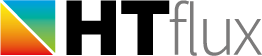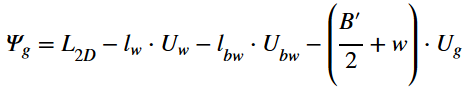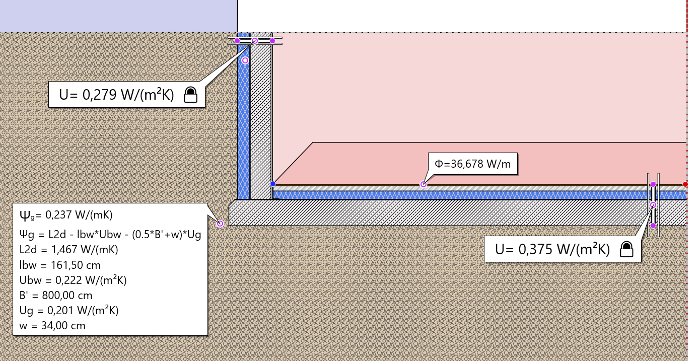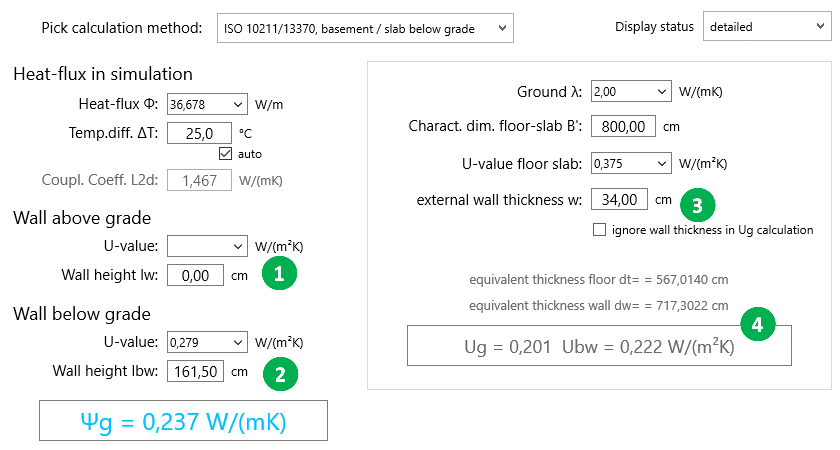# Ψg-calculation with slab below grade level (or heated basement) using ISO 10211 and ISO 13370

This version is very similar to the slab on grade calculation (ISO) method. The only difference is that the wall will be split into two parts. One above grade level and one below. Therefore you have to provide separate lengths as well as U-value for each segment.
The Ψg value will then be calculated using the following formula:The additional term compared to the slab on grade calculation (ISO) method represents the segment of the wall below grade level. Similar to the Ug value for the floor the U-value of the basement wall cannot be used directly. It has to be adapted to the so called Ubw value to also consider the heat flow through the soil. Similar to the Ug value HTflux will do this calculation for you, based on the methods described in ISO 13370.

### The wall thickness and slab-width issue

Unlike the floor on slab case, where the thickness of the wall us commonly not included in the calculation (set to 0) the wall thickness is still often considered for basements. In this case the wall thickness has to be provided here for an external dimensions reference. Even in this case ISO 13370 suggests to draw your model with a slab width of B’/2 measured from the internal side of the wall (even for the external dimension reference system). As this method described in ISO 13370 seems to be inconsistent with the external measurement system some users ignore this suggestion: they draw the model with a width B’/2 to the external edge of the wall and use a wall thickness of “0” for this calculation also.  Again: you should always apply the dimensions as they are defined in your buildings energy performance calculation method. This way the Ψ values will represent the correct compensation values to provide valid total heat flux numbers.

Depending on your task it will often be sufficient to start the model at the grade level. This means you can start the objects at ground level – or cover them with a rectangle set as “adiabatic”.  In this case you can leave the input fields for the wall above grade empty, or provide a height of “0”.
This example is shown here:If you choose this type of calculation the Ψg dialog will look like this:1. Enter the data for the wall above grade level here. If your model consists of the wall below surface only set the height input to 0 or leave it empty.
2. Enter the data for the wall below surface here. The U-value of the basement wall will be the base for the calculation of the Ubw value. Make sure the height provided is inline with your reference system.
3. If you are doing an external measurements calculation you can provide the total wall thickness here (including exterior insulation). In this case your model should have a total width of (B’/2 + w) measured to the exterior side of the basement wall. (more on this topic on the top of this page).
4. The U-values corrected with the “ground effect” are displayed here. Ug for the floor slab and Ubw for the wall segment below surface (or basement wall).

Regarding the other inputs, not marked with bullets, please refer to the slab on grade calculation (ISO) method page.